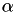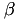Simpact Cyan Population based event driven simulation using mNRM
GslRandomNumberGenerator Class Reference

This class allows you to generate random numbers, and uses the GNU Scientific Library for this. More...

#include <gslrandomnumbergenerator.h>

## Public Member Functions

GslRandomNumberGenerator ()
Initialize the random number generator with a random seed, A specific seed can still be forced by setting the MNRM_DEBUG_SEED environment variable (can be useful for testing purposes). More...

GslRandomNumberGenerator (int seed)
Initialize the random number generator with a specific seed. More...

double pickRandomDouble ()
Generate a random floating point number in the interval [0,1]. More...

int pickRandomInt (int min, int max)
Chooses a random number from min to max (both are included). More...

unsigned int pickPoissonNumber (double lambda)
Chooses a random number according to the Poisson distribution with parameter lambda. More...

unsigned int pickBinomialNumber (double p, unsigned int n)
Pick a random number from the binomial distribution, for n trials with a probability of success p for each trial. More...

double pickGaussianNumber (double mean, double sigma)
Picks a random number from the gaussian distribution with parameters mean and sigma. More...

double pickBetaNumber (double a, double b)
Pick a random number from a beta distribution with a and b as values forandrespectively. More...

double pickWeibull (double lambda, double kappa)
Picks a random number from a Weibull distribution with specified parameters. More...

double pickWeibull (double lambda, double kappa, double ageMin)
Picks a random number from a distribution which has a Weibull shape with specified parameters above ageMin, and which is zero below that age. More...

double pickLogNorm (double zeta, double sigma)
Picks a random number from a log-normal distribution with parameters zeta and sigma. More...

double pickGamma (double a, double b)
Picks a random number from the gamma distribution with a and b defined as in the formula prob(x) = x^(a-1)*exp(-x/b)/(b^a * Gamma(a)) . More...

std::pair< double, double > pickBivariateGaussian (double muX, double muY, double sigmaX, double sigmaY, double rho)
Picks a random number from a two dimensional gaussian distribution with specified parameters (rho is the correlation coefficient). More...

## Detailed Description

This class allows you to generate random numbers, and uses the GNU Scientific Library for this.

## Constructor & Destructor Documentation

 GslRandomNumberGenerator::GslRandomNumberGenerator ( )

Initialize the random number generator with a random seed, A specific seed can still be forced by setting the MNRM_DEBUG_SEED environment variable (can be useful for testing purposes).

 GslRandomNumberGenerator::GslRandomNumberGenerator ( int seed )

Initialize the random number generator with a specific seed.

## Member Function Documentation

 double GslRandomNumberGenerator::pickBetaNumber ( double a, double b )

Pick a random number from a beta distribution with a and b as values forandrespectively.

 unsigned int GslRandomNumberGenerator::pickBinomialNumber ( double p, unsigned int n )

Pick a random number from the binomial distribution, for n trials with a probability of success p for each trial.

 std::pair< double, double > GslRandomNumberGenerator::pickBivariateGaussian ( double muX, double muY, double sigmaX, double sigmaY, double rho )

Picks a random number from a two dimensional gaussian distribution with specified parameters (rho is the correlation coefficient).

 double GslRandomNumberGenerator::pickGamma ( double a, double b )

Picks a random number from the gamma distribution with a and b defined as in the formula prob(x) = x^(a-1)*exp(-x/b)/(b^a * Gamma(a)) .

 double GslRandomNumberGenerator::pickGaussianNumber ( double mean, double sigma )

Picks a random number from the gaussian distribution with parameters mean and sigma.

 double GslRandomNumberGenerator::pickLogNorm ( double zeta, double sigma )

Picks a random number from a log-normal distribution with parameters zeta and sigma.

 unsigned int GslRandomNumberGenerator::pickPoissonNumber ( double lambda )

Chooses a random number according to the Poisson distribution with parameter lambda.

 double GslRandomNumberGenerator::pickRandomDouble ( )

Generate a random floating point number in the interval [0,1].

 int GslRandomNumberGenerator::pickRandomInt ( int min, int max )

Chooses a random number from min to max (both are included).

 double GslRandomNumberGenerator::pickWeibull ( double lambda, double kappa )

Picks a random number from a Weibull distribution with specified parameters.

 double GslRandomNumberGenerator::pickWeibull ( double lambda, double kappa, double ageMin )

Picks a random number from a distribution which has a Weibull shape with specified parameters above ageMin, and which is zero below that age.

The documentation for this class was generated from the following files: